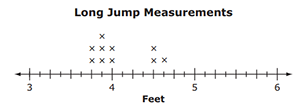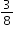# MAFS.4.MD.2.4

Make a line plot to display a data set of measurements in fractions of a unit (1/2, 1/4, 1/8). Solve problems involving addition and subtraction of fractions by using information presented in line plots. For example, from a line plot find and interpret the difference in length between the longest and shortest specimens in an insect collection.
General Information
Subject Area: Mathematics
Domain-Subdomain: Measurement and Data
Cluster: Level 2: Basic Application of Skills & Concepts
Cluster: Represent and interpret data. (Supporting Cluster) -

Clusters should not be sorted from Major to Supporting and then taught in that order. To do so would strip the coherence of the mathematical ideas and miss the opportunity to enhance the major work of the grade with the supporting clusters.

Date of Last Rating: 02/14
Status: State Board Approved
Assessed: Yes
Test Item Specifications

• Assessment Limits :
Measurement units are limited to halves, quarters, and eighths. Addition and subtraction of fractions is limited to fractions with like denominators. Limit addition and subtraction to solutions within 1,000.
• Calculator :

No

• Context :

Allowable

Sample Test Items (1)
• Test Item #: Sample Item 1
• Question: A line plot with long jump data is given.Allison jumpedfoot shorter than the farthest jump.

How far, in feet, did Allison jump?

• Difficulty: N/A
• Type: EE: Equation Editor

## Related Courses

This benchmark is part of these courses.
5012060: Mathematics - Grade Four (Specifically in versions: 2014 - 2015, 2015 - 2022 (current), 2022 and beyond)
5020050: Science - Grade Four (Specifically in versions: 2014 - 2015, 2015 - 2022 (current), 2022 and beyond)
5021060: Social Studies Grade 4 (Specifically in versions: 2014 - 2015, 2015 - 2022 (current), 2022 - 2023, 2023 and beyond)
7712050: Access Mathematics Grade 4 (Specifically in versions: 2014 - 2015, 2015 - 2018, 2018 - 2022 (current), 2022 and beyond)
7720050: Access Science Grade 4 (Specifically in versions: 2014 - 2015, 2015 - 2018, 2018 and beyond (current))
5020110: STEM Lab Grade 4 (Specifically in versions: 2016 - 2022 (current), 2022 and beyond)
5012065: Grade 4 Accelerated Mathematics (Specifically in versions: 2019 - 2022 (current), 2022 and beyond)
5012015: Foundational Skills in Mathematics 3-5 (Specifically in versions: 2019 - 2022 (current), 2022 and beyond)

## Related Access Points

Alternate version of this benchmark for students with significant cognitive disabilities.
MAFS.4.MD.2.AP.4a: Solve problems involving addition and subtraction of fractions with like denominators (2, 4, and 8) by using information presented in line plots.

## Related Resources

Vetted resources educators can use to teach the concepts and skills in this benchmark.

## Assessments

Sample 3 - Fourth Grade Math State Interim Assessment:

This is a State Interim Assessment for fourth grade.

Type: Assessment

Sample 2 - Fourth Grade Math State Interim Assessment:

This is a State Interim Assessment for fourth grade.

Type: Assessment

## Formative Assessments

Science Experiment Part Two:

Students are asked to analyze data presented in a line plot and solve problems related to the data.

Type: Formative Assessment

Science Experiment Part One:

Students are asked to use a given set of data to create a line plot with an appropriate scale.

Type: Formative Assessment

Race Cars Part Two:

Students are asked to analyze data presented in a line plot and solve problems related to the data.

Type: Formative Assessment

Race Cars Part One:

Students are asked to use a given set of data to create a line plot with an appropriate scale.

Type: Formative Assessment

## Lesson Plans

Going Green - Renewable and Non-Renewable Florida Resources:

Students will use their knowledge of renewable and non-renewable resources to create a green community in Florida. The students will be given a map of a plot of land in Florida on which to develop a green community. They will need to use renewable resources while protecting the non-renewable resources on the plot of land.

Type: Lesson Plan

One Leg Up:

In this lesson the students will be using a line plot to display a data set of measurements in fractions of a unit (1/2, 1/4, 1/8). They will be measuring different lengths of insects, recording the lengths, and then plotting the data on a line plot. From this data they will solve problems involving the addition and subtraction of fractions.

Type: Lesson Plan

Marshmallow Math:

In this lesson, students are physically engaged in measuring distances of tossed marshmallows to the nearest 1/2 foot. Using their measurements, they will represent the data on a line plot and then solve word problems involving addition and subtraction of mixed numbers. This is a fun lesson that motivates students to become excited about the difficult world of fractions.

Type: Lesson Plan

## Original Student Tutorial

Logging Lengths with Line Plots:

Learn how to create a line plot and analyze data in the line plot in this interactive tutorial.  You will also see how to add and subtract using the line plot to solve problems based on the line plots.

Type: Original Student Tutorial

## Virtual Manipulative

KidsZone: Create a Graph:

Create bar, line, pie, area, and xy graphs.

Type: Virtual Manipulative

## MFAS Formative Assessments

Race Cars Part One:

Students are asked to use a given set of data to create a line plot with an appropriate scale.

Race Cars Part Two:

Students are asked to analyze data presented in a line plot and solve problems related to the data.

Science Experiment Part One:

Students are asked to use a given set of data to create a line plot with an appropriate scale.

Science Experiment Part Two:

Students are asked to analyze data presented in a line plot and solve problems related to the data.

## Original Student Tutorials Mathematics - Grades K-5

Logging Lengths with Line Plots:

Learn how to create a line plot and analyze data in the line plot in this interactive tutorial.  You will also see how to add and subtract using the line plot to solve problems based on the line plots.

## Student Resources

Vetted resources students can use to learn the concepts and skills in this benchmark.

## Original Student Tutorial

Logging Lengths with Line Plots:

Learn how to create a line plot and analyze data in the line plot in this interactive tutorial.  You will also see how to add and subtract using the line plot to solve problems based on the line plots.

Type: Original Student Tutorial

## Parent Resources

Vetted resources caregivers can use to help students learn the concepts and skills in this benchmark.